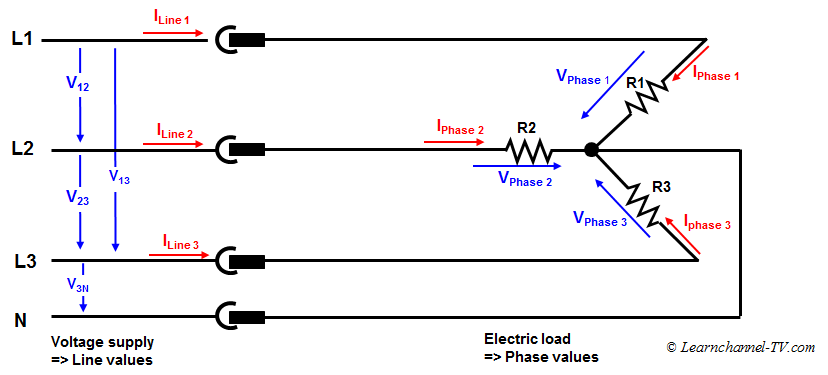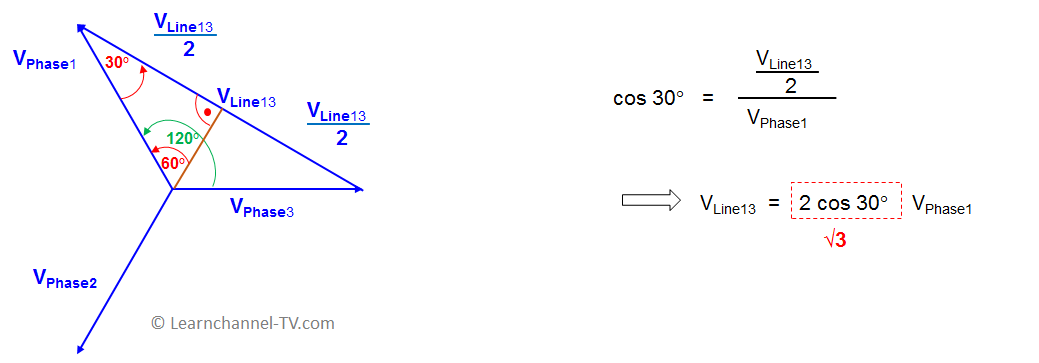(English)

## STAR Connection

The ends of the phases are connected together to form the so-called neutral- or star point. If all three resistors are the same size, the phase currents cancel each other out at the zero point and the neutral line can be omitted.3 Phase Current - Star-connection - Voltage and Current values

What you can see from the circuit:

In Star connection the line current is equal to the phase current:   ILine =  IPhase

What about the voltage? The relation between the Line voltage and the Phase voltage can be derived from the pointer diagram:Star connection - Relation between Line voltage and Phase Voltage

In star Connection the Line Voltage is √3 times of Phase Voltage:  VLine = √3 VPhase